# Pipe cross section

The pipe has an outside diameter 1100 mm and the pipe wall is 100 mm thick. Calculate the cross section of this pipe.

Correct result:

S =  6361.7251 cm2

#### Solution: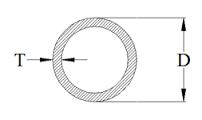We would be pleased if you find an error in the word problem, spelling mistakes, or inaccuracies and send it to us. Thank you!#### You need to know the following knowledge to solve this word math problem:

We encourage you to watch this tutorial video on this math problem:

## Next similar math problems:

• Two circlesTwo circles with a radius 4 cm and 3 cm have a center distance 0.5cm. How many common points have these circles?
• GardenThe garden around the new majer is divided as follows: 35% vegetable, 30% fruit orchards, 10% flowers and the remaining 120 m2 are lawns. What is the total area of the garden?
• Trapezium 2Trapezium has an area of 24 square cms. How many different trapeziums can be formed ?
• Circle area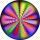Calculate the circle area with a radius of 1.2 m.
• MineWheel in traction tower has a diameter 4 m. How many meters will perform an elevator cabin if wheel rotates in the same direction 89 times?
• 22/7 circleCalculate approximately area of a circle with radius 20 cm. When calculating π use 22/7.
• An equilateral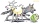An equilateral triangle with a side 10 m represents a wooden platform standing in a lawn. A goat is tied to a corner with a 15 m rope. What is the maximum amount of grazing area available to the goat?
• Calculate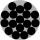Calculate the area of a circle whose radius is 5 dm.
• CD disc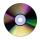A compact disc has a diameter of 11.8 cm. What is the surface area of the disc in square centimeters?
• FlowerbedIn the park there is a large circular flowerbed with a diameter of 12 m. Jakub circulated him ten times and the smaller Vojtoseven times. How many meters each went by and how many meters did Jakub run more than Vojta?
• Circle - simpleCalculate the area of a circle in dm2, if its circumference is 31.4 cm.
• SidewalkThe city park is a circular bed of flowers with a diameter of 8 meters, around it the whole length is 1 meter wide sidewalk . What is the sidewalk area?
• RhombusPQRS is a rhombus. Given that PQ=3 cm & height of rhombus is given 2 cm. Calculate its area.
• LawnsBefore a sports hall are two equally large rectangular lawns, each measuring 40 m and 12 m. Maintenance of 10m² lawn cost 45 CZK yearly. On each lawn is circular flowerbed with a diameter 8 meters. How much money is needed each year to take on lawn care?
• CableCable consists of 8 strands, each strand consists of 12 wires with diameter d = 0.5 mm. Calculate the cross-section of the cable.
• Diameter to areaFind the area of a circle whose diameter is 26cm.
• DiameterWhat is the inside diameter of the cylinder container and if half a liter of water reaches a height 15 cm?